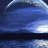## 雷锦志的个人博客分享http://blog.sciencenet.cn/u/jzlei乱七八糟的随想录

• 天津工大，研究员

• 数理科学->数学->应用数学方法

扫一扫，分享此博客主页lzmaks 2017-3-17 22:10
Collatz 猜想的证明： 构造整域变换：x=3n+d,y=3n-d,z=n/2。 1. d=0,n=0,x=3×0+0=0,y=3×0-0=0,z=0/2=0。2.d=1,n=0,x=3×0+1=1,y=3×0-1=-1,z=0/2=0。3.当d属于Z,n属于N+时，x=3×1+d,y=3×1-d,z=n/2；当d属于Z,n属于N-时,x=3×(-1)+d,y=3×(-1)-d,z=n/2。(1)d属于Z,n属于N+。【1】(3×1+d1-1)/3=(3×1-d1)/2,d1=1;(3×1-d2-1)/3=(3×1+d2)/2,d2=-1。【2】(3×1-d3)/2=2(3×1+d3),d3=9/5;(3×1+d4)/2=2(3×1-d4),d4=-9/5。【3】3(3×1-d5)+1=2(3×1+d5),d5=4/5;3(3×1+d6)+1=2(3×1-d6),d6=-4/5。取d=1,则x=4,y=2,可得循环圈A=(4,2,1,4 ... ...beijing2008ch 2016-12-2 11:06juzizhou 2014-2-12 20:09

Systems Biology--Modelling, Analysis, and Simulation 2021-06-12

(1311)次阅读|(0)个评论

(8043)次阅读|(0)个评论

(2475)次阅读|(0)个评论

(3914)次阅读|(0)个评论

国庆节审一篇 IEEE/TCBB 的稿子，其烂无比，糟点太多，忍不住要吐槽。 1.  平均每两句话就有一句是有语法错误的，能看下去基本靠猜。作者是两 ...
(4355)次阅读|(1)个评论

暑假给几个学生讲授了一周的系统生物学短期课程，主要目的是为初学者介绍一点生物学的基础知识和数学建模与分析基础。其中有几次课有录像。做个记录分享 ...
(4807)次阅读|(0)个评论

故事起源于电视科教频道中介绍一些动物为了繁衍后代而牺牲自己的无私行为。 在动物世界中，有一些动物为了繁衍后代而付出自己的生命，例如螳螂，狼蛛 ...
(2819)次阅读|(1)个评论

(5932)次阅读|(0)个评论

现在还没有记录

GMT+8, 2023-3-20 21:51